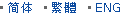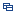| Recent Visit History | Join Audited Suppliers | Help | Region Guide
» » » » Nanjing Product List

91.

## Borofloat Glass Lens for Fiber Optics[Nov 20, 2020]

Meniscus lenses have one concave and one convex surface. For this lens type, the two curvatures are chosen to minimize the spherical aberration at one laser wavelength, hence ...

92.

## Optical Glass Bk7 Positive Lens[Nov 20, 2020]

Double convex lenses have two outward curved surfaces, normally the two surfaces have same radius of curvature, with finely polished. Bi convex lenses have positive focal length, ...

93.

## Optical Glass N-Sf11 Plano Convex Lens[Nov 20, 2020]

Plano convex lenses are designed to focus and collimate the light in optical system. The lenses are often used for laser, fiber, medical, sensor, communication and surveying ...

94.

## Surveying Instrument Plano Concave Glass Lens[Nov 20, 2020]

Plano-concave lenses are thicker at the edge than in the center and flat on another surface. Plano concave lens (PCV) has negative focal length. So the lenses are used to bend ...

95.

## Plano Convex Lens Optical Glass Double Convex Lens[Nov 20, 2020]

Double convex lenses have two outward curved surfaces, normally the two surfaces have same radius of curvature, with finely polished. Bi convex lenses have positive focal length, ...

96.

## Optical Bk7 Glass Double Convex Lens[Nov 20, 2020]

Double convex lenses comprise two convex spherical surfaces, generally with the same radius of curvature. Bi convex lenses are used as magnifiers, condensing lenses and ...

97.

## Anti Reflective Coated Plano Convex Lens Optical Glass[Nov 20, 2020]

Plano convex lenses are designed to focus and collimate the light in optical system. The lenses are often used for laser, fiber, medical, sensor, communication and surveying ...

98.

## Surveying Level Optical Glass Lens[Nov 20, 2020]

Double convex lenses have two outward curved surfaces, normally the two surfaces have same radius of curvature, with finely polished. Bi convex lenses have positive focal length, ...

99.

## Custom Made Optical Glass Meniscus Lens[Nov 20, 2020]

Meniscus lenses have one concave and one convex surface. For this lens type, the two curvatures are chosen to minimize the spherical aberration at one laser wavelength, hence ...

100.

## Optical Glass Plano Convex Lens Custom Made[Nov 20, 2020]

Plano convex lenses are designed to focus and collimate the light in optical system. The lenses are often used for laser, fiber, medical, sensor, communication and surveying ...

101.

## Projector Bi Convex Lens Optical Glass[Nov 20, 2020]

Double convex lenses have two outward curved surfaces, normally the two surfaces have same radius of curvature, with finely polished. Bi convex lenses have positive focal length, ...

102.

## Condensing Optical Glass Double Convex Lens[Nov 20, 2020]

Double convex lenses comprise two convex spherical surfaces, generally with the same radius of curvature. Bi convex lenses are used as magnifiers, condensing lenses and ...

103.

## Ar Coated Optical Glass Plano Convex Lens[Nov 20, 2020]

Plano convex lenses are designed to focus and collimate the light in optical system. The lenses are often used for laser, fiber, medical, sensor, communication and surveying ...

104.

## Surveying Level Optical Glass Plano Convex Lens[Nov 20, 2020]

Double convex lenses have two outward curved surfaces, normally the two surfaces have same radius of curvature, with finely polished. Bi convex lenses have positive focal length, ...

105.

## Optical Glass Bk7 Scanning Lens[Nov 20, 2020]

Double convex lenses have two outward curved surfaces, normally the two surfaces have same radius of curvature, with finely polished. Bi convex lenses have positive focal length, ...

Hot Region Index: ABCDEFGHIJKLMNPQRSTUWXYZ